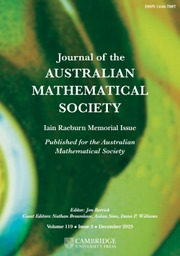Home
Hostname: page-component-8bbf57454-nshs2 Total loading time: 0.473 Render date: 2022-01-26T05:26:16.076Z Has data issue: true Feature Flags: { "shouldUseShareProductTool": true, "shouldUseHypothesis": true, "isUnsiloEnabled": true, "metricsAbstractViews": false, "figures": true, "newCiteModal": false, "newCitedByModal": true, "newEcommerce": true, "newUsageEvents": true }Journal of the Australian Mathematical Society

THE SMOOTHNESS OF ORBITAL MEASURES ON NONCOMPACT SYMMETRIC SPACES

Published online by Cambridge University Press:  26 April 2021

Abstract

Let$G/K$ be an irreducible symmetric space, where G is a noncompact, connected Lie group and K is a compact, connected subgroup. We use decay properties of the spherical functions to show that the convolution product of any$r=r(G/K)$ continuous orbital measures has its density function in$L^{2}(G)$ and hence is an absolutely continuous measure with respect to the Haar measure. The number r is approximately the rank of$G/K$. For the special case of the orbital measures,$\nu _{a_{i}}$, supported on the double cosets$Ka_{i}K$, where$a_{i}$ belongs to the dense set of regular elements, we prove the sharp result that$\nu _{a_{1}}\ast \nu _{a_{2}}\in L^{2},$ except for the symmetric space of Cartan class$AI$ when the convolution of three orbital measures is needed (even though$\nu _{a_{1}}\ast \nu _{a_{2}}$ is absolutely continuous).

MSC classification

Type
Research Article
© 2021 Australian Mathematical Publishing Association Inc

Access options

Get access to the full version of this content by using one of the access options below. (Log in options will check for institutional or personal access. Content may require purchase if you do not have access.)

Footnotes

This research is supported in part by NSERC 2016-03719 and by Sultan Qaboos University. The authors thank Acadia University for their hospitality when this research was done.

References

Abramowitz, M. and Stegun, I., Handbook of Mathematical Functions, National Bureau of Standards Applied Mathematics Series, 55 (U.S. Government Printing Office, Washington, DC, 1970).Google Scholar
Anchouche, B., ‘Regularity of the Radon–Nikodym derivative of a convolution of orbital measures on noncompact symmetric spaces’, Preprint, 2018.10.4310/AJM.2018.v22.n2.a1CrossRefGoogle Scholar
Anchouche, B., Gupta, S. K. and Plagne, A., ‘Orbital measures on$\mathrm{SU}(2)/\mathrm{SO}(2)$’, Monatsh. Math. 178 (2015), 493520.10.1007/s00605-015-0812-xCrossRefGoogle Scholar
Anker, J. P., ‘The spherical Fourier transform of rapidly decreasing functions. A simple proof of a characterization due to Harish-Chandra, Helgason, Trombi and Varadarajan’, J. Funct. Anal. 96 (1991), 331349.10.1016/0022-1236(91)90065-DCrossRefGoogle Scholar
Caselle, M. and Magnea, U., ‘Random matrix theory and symmetric spaces’, Phys. Rep. 394 (2004), 41156.10.1016/j.physrep.2003.12.004CrossRefGoogle Scholar
Duistermaat, J. J., Kolk, J. A. C. and Varadarajan, V. S., ‘Functions, flows and oscillatory integrals on flag manifolds and conjugacy classes in semisimple Lie groups’, Compos. Math. 49 (1983), 309398.Google Scholar
Fitouhi, A. and Hamza, M., ‘A uniform expansion for the eigenfunction of a singular second-order differential operator’, SIAM J. Math. Anal. 21 (1990), 16191632.10.1137/0521088CrossRefGoogle Scholar
Graczyk, P. and Sawyer, P., ‘Absolute continuity of convolutions of orbital measures on Riemannian symmetric spaces’, J. Funct. Anal. 259 (2010), 17591770.10.1016/j.jfa.2010.05.017CrossRefGoogle Scholar
Graczyk, P. and Sawyer, P., ‘A sharp criterion for the existence of the product formula on symmetric spaces of type${A}_n$’, J. Lie Theory 20 (2010), 751766.Google Scholar
Graczyk, P. and Sawyer, P., ‘Convolution of orbital measures on symmetric spaces of type${C}_p$ and${D}_p$’, J. Aust. Math. Soc. 98 (2015), 232256.10.1017/S1446788714000494CrossRefGoogle Scholar
Graczyk, P. and Sawyer, P., ‘Convolution of orbital measures on symmetric spaces: a survey’, Contemp. Math. 668 (2016), 81110.10.1090/conm/668/13398CrossRefGoogle Scholar
Gupta, S. K. and Hare, K. E., ‘${L}^2$-singular dichotomy for orbital measures of classical compact Lie groups’, Adv. Math. 222 (2009), 15211573.10.1016/j.aim.2009.06.008CrossRefGoogle Scholar
Gupta, S. K. and Hare, K. E., ‘The absolute continuity of convolutions of orbital measures in symmetric spaces’, J. Math. Anal. Appl. 450 (2017), 81111.10.1016/j.jmaa.2017.01.027CrossRefGoogle Scholar
Gupta, S. K., Hare, K. E. and Seyfaddini, S., ‘${L}^2$-singular dichotomy for orbital measures of classical simple Lie algebras’, Math. Z. 262 (2009), 91124.10.1007/s00209-008-0364-zCrossRefGoogle Scholar
Hare, K. E. and Skoufranis, P., ‘The smoothness of orbital measures on exceptional Lie groups and algebras’, J. Lie Theory 21 (2011), 9871007.Google Scholar
Hare, K. E., Wilson, D. and Yee, W. L., ‘Pointwise estimates of the size of characters of compact Lie groups’, J. Aust. Math. Soc. 69 (2000), 6184.10.1017/S1446788700001841CrossRefGoogle Scholar
Hare, K. E. and Yeats, K., ‘The size of characters of exceptional Lie groups’, J. Aust. Math. Soc. 77 (2004), 233248.10.1017/S1446788700013604CrossRefGoogle Scholar
Helgason, S., Differential Geometry, Lie Groups and Symmetric Spaces (Academic Press, New York, 1978).Google Scholar
Helgason, S., Groups and Geometric Analysis (Academic Press, Orlando, FL, 1984).Google Scholar
Humphreys, J., Introduction to Lie Algebras and Representation Theory (Springer, New York, 1994).Google Scholar
Ragozin, D., ‘Zonal measure algebras on isotropy irreducible homogeneous spaces’, J. Funct. Anal. 17 (1974), 355376.10.1016/0022-1236(74)90046-9CrossRefGoogle Scholar
Wolf, J., ‘Harmonic Analysis on Commutative Spaces’, AMS Mathematical Surveys and Monographs, 142 (American Mathematical Society, Providence, RI, 2007).10.1090/surv/142CrossRefGoogle Scholar

Send article to Kindle

Note you can select to send to either the @free.kindle.com or @kindle.com variations. ‘@free.kindle.com’ emails are free but can only be sent to your device when it is connected to wi-fi. ‘@kindle.com’ emails can be delivered even when you are not connected to wi-fi, but note that service fees apply.

Find out more about the Kindle Personal Document Service.

THE SMOOTHNESS OF ORBITAL MEASURES ON NONCOMPACT SYMMETRIC SPACES
Available formats
×

Send article to Dropbox

To send this article to your Dropbox account, please select one or more formats and confirm that you agree to abide by our usage policies. If this is the first time you use this feature, you will be asked to authorise Cambridge Core to connect with your <service> account. Find out more about sending content to Dropbox.

THE SMOOTHNESS OF ORBITAL MEASURES ON NONCOMPACT SYMMETRIC SPACES
Available formats
×

To send this article to your Google Drive account, please select one or more formats and confirm that you agree to abide by our usage policies. If this is the first time you use this feature, you will be asked to authorise Cambridge Core to connect with your <service> account. Find out more about sending content to Google Drive.

THE SMOOTHNESS OF ORBITAL MEASURES ON NONCOMPACT SYMMETRIC SPACES
Available formats
×
×